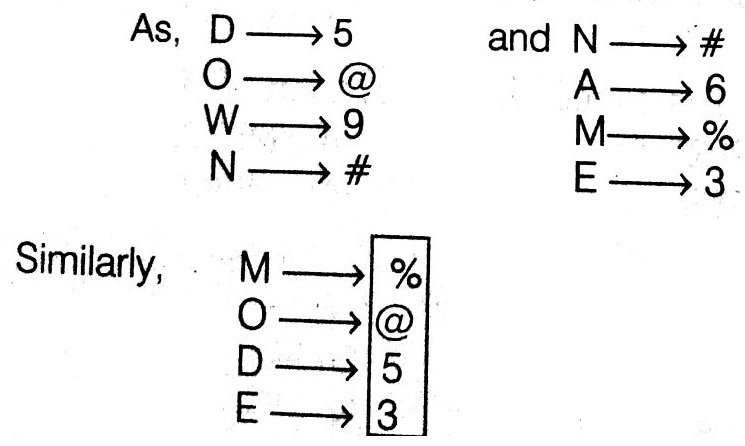• Save

# Symbol - Coding Decoding Questions

3 months ago 543 Views
NEW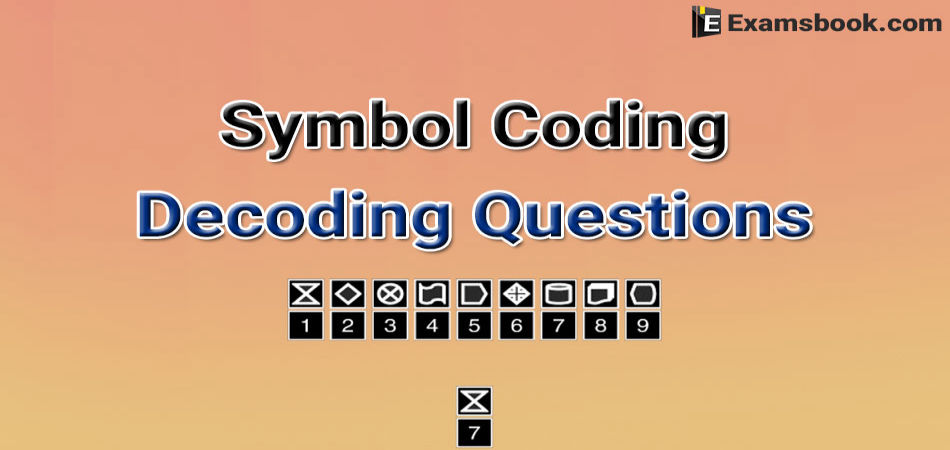In this type of questions, you have to give the answers according to given symbol coding. To understand these symbol coding decoding questions, you can practice with these important symbol questions for competitive exams which are below:

After reading symbol coding questions, you can practice of Deciphering Coding Questions with answers for your better performance.

## Symbol Coding Questions with Solutions

Q.1. In a certain code, RAID is written as %#*$, RIPE is written as % * @ ©. How is DEAR written up in that code? (A)$ * 37

(B) 3$7* (C)$73*

(D) $37* Ans . D According to the question, Letters R A I D R I P E Symbol code % # *$ % * @ ©

Hence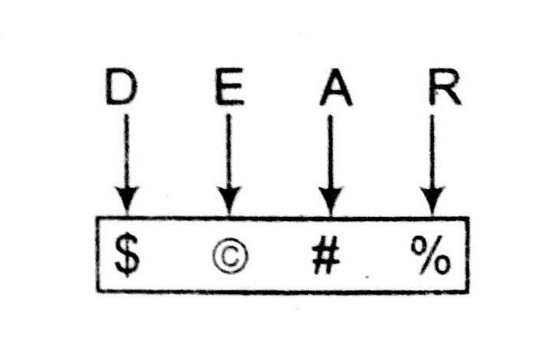Q.2. In a certain code, MODERN is written as 5%38#@ and WIN is written as 6©@. How is RIDE Written in that code?

(h) # @38

Ans . A

According to the question

Letter                M O D E R N W I   N

Symbol Code    5  % 3 8  # @ 6 © @

Hence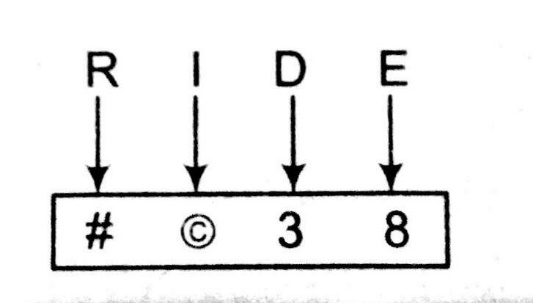Q.3. In a certain code, ROPE is written as %57$, DOUBT is written as 35#8* and LIVE is written as @24$. How is TROUBLE written in that code?

(a) *%5#8@$(b) *%#58@$

(c) *%5#8@4

(d) *%#58$@ Ans . A According to the question Letters R O P E D O U B T L I V E Symbol code % 5 7$ 3 5 # 8 * @ 2 4 $Hence,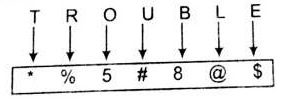Q.4. In a certain code, MEAN is written as$57* and DOME is written as 93$5. How is MOAN written in that code? (a)$ * 37

(b) 3$7* (c)$73 *

(d) $37* Ans . D According to the question Letters M E A N D O M E Symbol code$  5 7  *  9 3  $5 Hence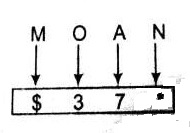Q.5. In a certain code, 'BOY' is written as '$*•' and 'HOUR' is written as ‘@*£O’. How is 'RUBY' written in that code?

(a) O£$• (b) O$£

(c) • £$O (d) •$£O

Ans .   A

According to the question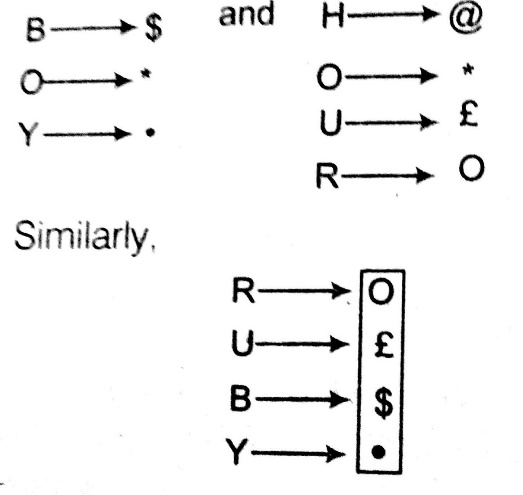Q.6. In a certain code, ‘DOWN’ is written as ‘5@9#’ and ‘NAME’ is written as ‘#6%3’. How would ‘MODE’ be written in that code?

(A) %653

(B) %@63

(C) %5@3

(D) %@53

Ans .   D